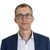# App Development

Announcements
Now Live: Qlik Sense SaaS Simplified Authoring – Analytics Creation for Everyone: READ DETAILS
cancel
Showing results for
Did you mean:Contributor

## Converting Pixels to Lat/Lon for Floorplan in QlikSense

Hello everyone,

I have followed Christof in his tutorial of building Office Floor Plans (Qlik Sense Office Floor Plans with native GeoObject), and is able to replicate his work with Point layer. Now on my dashboard, I would like to switch to a different layer (Layer/Area) instead of (Layer/Point) to draw an area on the map to highlight an item (for e.g. vending machine or table) on the floor plan. I understand that in order to draw an area, we need four coordinates, i.e. [[x,y],[x2,y2],[x3,y3],[x4,y4]]. I have included all four coordinates of the item in a table imported over to the dashboard.

The units for these coordinates are in pixels measured using Irfanview and looks like this:
Item | X | Y | X2 | Y2 | X3 | Y3 | X4 | Y4
Table | 30 | 98 | 90 | 100 | 30 | 120 | 90 | 120
Vending Machine | 1475 | 99 | 1536 | 99 | 1475 | 121 | 1536 | 121
Using these values, I have defined a new dimension ("Area_Coordinates") that will include these four pairs of values in the following format: "[[x,y],[x2,y2],[x3,y3],[x4,y4]]". Area_Coordinates, the new dimension, will then be placed in the Data of the Area Layer.

My background image's current size in pixels is 1570 x 265, and is using the projection of user defined (metres) in map settings as the video defines. However, the problem here is the map projection that I am unable to see anything highlighted. According to the image link in Christof's Github project link, I am supposed to convert the coordinates pixel to Lat/Lon projection? I have been trying this conversion in the Area_Coordinates but have been unsuccessful (I cant see any highlighted/coloured box). Does anyone has any good ideas to resolve this?

Some examples of formula I have tried:
1) //https://gis.stackexchange.com/questions/228489/how-to-convert-image-pixel-to-latitude-and-longitude
//mx = C + px * A
//my = F + py * D
='[[' & Round((RangeMax(X,Y)/2 - X/2) * 40075014 / RangeMax(X,Y) - 20037507) & ',' & Round((RangeMax(X,Y)/2 - Y/2) * -40075014 / RangeMax(X,Y) + 20037507) & '],'
& '[' & Round((RangeMax(X2,Y2)/2 - X2/2) * 40075014 / RangeMax(X2,Y2) - 20037507) & ',' & Round((RangeMax(X2,Y2)/2 - Y2/2) * -40075014 / RangeMax(X2,Y2) + 20037507) & '],'
& '[' & Round((RangeMax(X3,Y3)/2 - X3/2) * 40075014 / RangeMax(X3,Y3) - 20037507) & ',' & Round((RangeMax(X3,Y3)/2 - Y3/2) * -40075014 / RangeMax(X3,Y3) + 20037507) & '],'
& '[' & Round((RangeMax(X4,Y4)/2 - X4/2) * 40075014 / RangeMax(X4,Y4) - 20037507) & ',' & Round((RangeMax(X4,Y4)/2 - Y4/2) * -40075014 / RangeMax(X4,Y4) + 20037507) & ']]'
2) //SET fX2Long = Round((RangeMax(\$2,\$3)/2 - \$2/2 + \$1) * 40075014 / RangeMax(\$2,\$3) - 20037507);
//SET fY2Lat = Round((RangeMax(\$2,\$3)/2- \$3/2 + \$1) * -40075014 / RangeMax(\$2,\$3) + 20037507);
\$(fX2Long(X, vWidth, vHeight))
\$(fY2Lat(Y, vWidth, vHeight))
='[[' & Round((RangeMax(W,L)/2- L/2 + X) * -40075014 / RangeMax(W,L) + 20037507) & ',' & Round((RangeMax(W,L)/2 - L/2 + Y) * 40075014 / RangeMax(W,L) - 20037507) & '],'
& '[' & Round((RangeMax(W,L)/2- L/2 + X2) * -40075014 / RangeMax(W,L) + 20037507) & ',' & Round((RangeMax(W,L)/2 - L/2 + Y2) * 40075014 / RangeMax(W,L) - 20037507) & '],'
& '[' & Round((RangeMax(W,L)/2- L/2 + X3) * -40075014 / RangeMax(W,L) + 20037507) & ',' & Round((RangeMax(W,L)/2 - L/2 + Y3) * 40075014 / RangeMax(W,L) - 20037507) & '],'
& '[' & Round((RangeMax(W,L)/2- L/2 + X4) * -40075014 / RangeMax(W,L) + 20037507) & ',' & Round((RangeMax(W,L)/2 - L/2 + Y4) * 40075014 / RangeMax(W,L) - 20037507) & ']]'
3) Adding GeoMakePoint to no. 2 -
='[[' & GeoMakePoint(Round((RangeMax(W,L)/2- L/2 + X) * -40075014 / RangeMax(W,L) + 20037507), Round((RangeMax(W,L)/2 - L/2 + Y) * 40075014 / RangeMax(W,L) - 20037507)) & '],'
& '[' & GeoMakePoint(Round((RangeMax(W,L)/2- L/2 + X2) * -40075014 / RangeMax(W,L) + 20037507), Round((RangeMax(W,L)/2 - L/2 + Y2) * 40075014 / RangeMax(W,L) - 20037507)) & '],'
& '[' & GeoMakePoint(Round((RangeMax(W,L)/2- L/2 + X3) * -40075014 / RangeMax(W,L) + 20037507), Round((RangeMax(W,L)/2 - L/2 + Y3) * 40075014 / RangeMax(W,L) - 20037507)) & '],'
& '[' & GeoMakePoint(Round((RangeMax(W,L)/2- L/2 + X4) * -40075014 / RangeMax(W,L) + 20037507), Round((RangeMax(W,L)/2 - L/2 + Y4) * 40075014 / RangeMax(W,L) - 20037507)) & ']]'

3 RepliesPartner - Creator II

Hello,

I had the same question and I solved it with this script:

Set vWitdh = 1350;
Set vHeight = 530;

Zone:
*,
'[[' & X1_calc & ',' & Y1_calc & '],[' & X3_calc & ',' & Y3_calc & '],[' & X4_calc & ',' & Y4_calc & '],[' & X2_calc & ',' & Y2_calc & ']]' as Area_Geometry;
Zone,
Area,
X1,
Round((RangeMax(\$(vWitdh),\$(vHeight))/2 - \$(vWitdh)/2 + X1) * 40075014 / RangeMax(\$(vWitdh),\$(vHeight)) - 20037507) as X1_calc,
Y1,
Round((RangeMax(\$(vWitdh),\$(vHeight))/2- \$(vHeight)/2 + Y1) * -40075014 / RangeMax(\$(vWitdh),\$(vHeight)) + 20037507) as Y1_calc,
X2,
Round((RangeMax(\$(vWitdh),\$(vHeight))/2 - \$(vWitdh)/2 + X2) * 40075014 / RangeMax(\$(vWitdh),\$(vHeight)) - 20037507) as X2_calc,
Y2,
Round((RangeMax(\$(vWitdh),\$(vHeight))/2- \$(vHeight)/2 + Y2) * -40075014 / RangeMax(\$(vWitdh),\$(vHeight)) + 20037507) as Y2_calc,
X3,
Round((RangeMax(\$(vWitdh),\$(vHeight))/2 - \$(vWitdh)/2 + X3) * 40075014 / RangeMax(\$(vWitdh),\$(vHeight)) - 20037507) as X3_calc,
Y3,
Round((RangeMax(\$(vWitdh),\$(vHeight))/2- \$(vHeight)/2 + Y3) * -40075014 / RangeMax(\$(vWitdh),\$(vHeight)) + 20037507) as Y3_calc,
X4,
Round((RangeMax(\$(vWitdh),\$(vHeight))/2 - \$(vWitdh)/2 + X4) * 40075014 / RangeMax(\$(vWitdh),\$(vHeight)) - 20037507) as X4_calc,
Y4,
Round((RangeMax(\$(vWitdh),\$(vHeight))/2- \$(vHeight)/2 + Y4) * -40075014 / RangeMax(\$(vWitdh),\$(vHeight)) + 20037507) as Y4_calc
FROM [lib://WAG/CustomMaps/SharedWorkspace/sharedworkspace.xlsx]
(ooxml, embedded labels, table is Area)
Where
Corners = 4;Contributor
Author

Pwagner, thanks for sharing your solution. This does not actually work for me.Specialist

In case you did not find any solition - I was struggling with the same problem and could not get it to work. But finally I understood why:

The location field for the Area Layer in the map object will accept coordinates described as:

[[x1,y1],[x2,y2],[x3,y3],[x4,y4]]

however (as I became aware....) that what is longitude and latitude in the map object may not be what you expect. Try to opposite also:

[[y1,x1],[y2,x2],[y3,x3],[y4,x4]]

/Robert

Svebeck Consulting AB